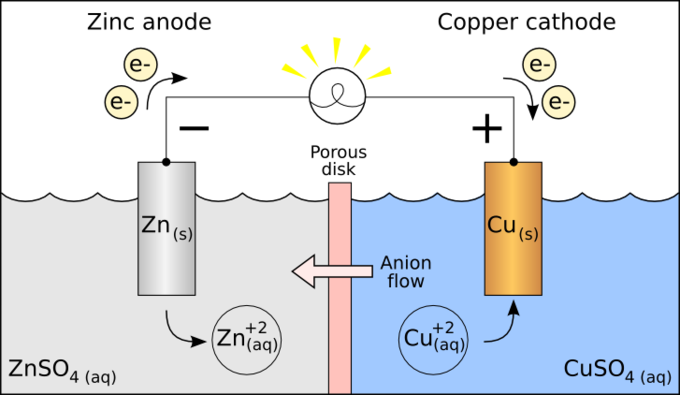## Equilibrium Constant and Cell Potential

#### Learning Objective

• Calculate the equilibrium constant, K, for a galvanic cell using the Nernst equation

#### Key Points

• In an electrochemical cell, each chemical species will move from areas with higher electrochemical potential to areas with lower electrochemical potential.
• The Nernst equation can be used to determine the equilibrium reduction potential of a half-cell in an electrochemical cell.
• The Nernst equation can also be used to determine the total voltage, or electromotive force, for a full electrochemical cell.

#### Term

• Nernst equationUsed to determine the equilibrium reduction potential of a half-cell in an electrochemical cell, as well as the total voltage for a full electrochemical cell.

## Electric and Chemical Potential

Each chemical species has an electrochemical potential (quantity with units of energy) at any given location, which represents how easy or difficult it is to add more of that species to that location. If possible, a species will move from areas with higher electrochemical potential to areas with lower electrochemical potential. In equilibrium, the electrochemical potential will be constant everywhere for each species. There might be a different value for each different species.

For example, if a glass of water has sodium ions (Na+) dissolved uniformly in it, and an electric field is applied across the water, then the sodium ions will tend to get pulled by the electric field toward one side. We say the ions have electric potential energy, and are moving to lower their potential energy.

Likewise, if a glass of water has a lot of dissolved sugar on one side and none on the other side, each sugar molecule will randomly diffuse around the water until there is and equal concentration of sugar everywhere. We say that the sugar molecules have a “chemical potential,” which is higher in the high-concentration areas, and the molecules move to lower their chemical potential.

These two examples show that an electrical potential and a chemical potential can both give the same result: a redistribution of the chemical species. Therefore, it makes sense to combine them into a single “potential,” the electrochemical potential, which can directly give the net redistribution by taking both into account.

## The Nernst Equation and Electrochemical Potential

In electrochemistry, the Nernst equation can be used, in conjunction with other information, to determine the equilibrium reduction potential of a half-cell. It can also be used to determine the total voltage, or electromotive force, for a full electrochemical cell. The Nernst equation gives a formula that relates the numerical values of the concentration gradient to the electrical gradient that balances it.

For example, let’s say a concentration gradient was established by dissolving KCl in half of a divided vessel that was originally full of H2O. A membrane permeable to K+ ions was introduced between the two halves. Empirically, an equilibrium situation would arise where the chemical concentration gradient could be balanced by an electrical gradient that opposes the movement of charge. In other words, a voltage could be applied to stop the movement of the K+ ions.

We can also consider an example where the solutions are CuSO4 and ZnSO4. Each solution has a corresponding metal strip in it, and a salt bridge or porous disk connecting the two solutions. This allows SO42- ions to flow freely between the copper and zinc solutions. In order to calculate the standard potential, we have to look up the half-reactions of copper and zinc.

The standard cell potential for the reaction is then +0.34 V – (-0.76 V) = +1.10 V. The polarity of the cell is determined by knowing that zinc metal is a stronger reducing agent than copper metal. Therefore, the standard reduction potential for zinc is more negative than that of copper. Thus, zinc metal will lose electrons to copper ions and develop a positive electrical charge.Galvanic cellSchematic of a galvanic cell for the reaction between Zn and Cu.

The cell equilibrium constant, K, can be derived from the Nernst equation:

$E=E^o−\frac{RT}{nF}lnQ$

In this equation, E is the cell potential, Eo is the standard cell potential (i.e., measured under standard conditions), F is Faraday’s constant, R is the universal gas constant, T is the temperature in degrees Kelvin, Q is the reaction quotient (which has the same algebraic from as the equilibrium constant expression, except it applies to any time during the reaction’s progress), and n is the number of moles of electrons that are transferred in the balanced chemical equation of the redox process.

The cell potential is zero at equilibrium (E=0), and Q (the reaction quotient) can now be designated as the equilibrium constant K. Solving for lnK, we obtain:

$ln\ K = \frac {nFE^o}{RT}$

## Example

Calculate the equilibrium constant K, from the following reaction studied at a temperature of 298K:

$Zn^{2+} + Cu \rightarrow Cu^{2+} + Zn \ \ \ \ \ \ \ \ \ \ \ \ \ \ \ E^o = 1.10\ V$

$ln\ K = \frac {nFE^o}{RT}$

In this problem, 2 moles of electrons are being transferred, F is 96485 $\frac {Coulombs}{mole}$, R is 8.31$\frac {Joules}{K \times mole}$, T is 298 K, and Eo is 1.10$\frac {Joules}{Coulomb}$.

Plugging the values into the equation, we get:

$ln\ K = \frac {2 \times 96485 \times 1.10}{8.31\times 298}$

$ln K = 85.7$

Solving for K, we get:

K = e85.7

K = 1.68 x 1037

This is a particularly high equilibrium constant value, indicating that the equilibrium strongly favors the formation of products (the reaction is effectively irreversible towards the formation of products).